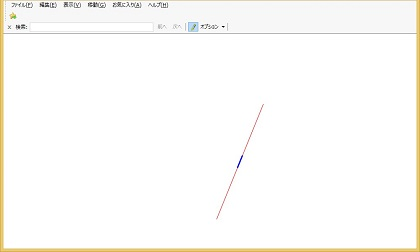# The endpoints of an arc may not be drawn correctly

This article helps you resolve the problem where starting point and endpoints may not be drawn in the correct position when you draw an arc that has a large radius.

Original product version:   .NET Framework 3.5 Service Pack 1, .NET Framework 3.5.1, .NET Framework 4.5
Original KB number:   2984519

## Symptoms

When you draw an arc with a large radius, the starting and finishing points of the arc may not be drawn correctly depending on the parameters set.

## Cause

On web browsers, such as Internet Explorer, it's possible to display graphics of WPF applications that load and display images represented in SVG (Scalable Vector Graphics) format, or applications that display images using Loose XAML (which is the function to load and render the markup without compiling).

The displaying of SVG images and the rendering engine of Loose XAML use DirectX (Direct3D9).

However, the processing inside DirectX (Direct3D9) is carried out using a single-precision floating-point number. Therefore, there's a possibility of unexpected image to be drawn if you use a large number that exceeds the limit of the processing.

For example, in Loose XAML, when you draw an arc that has a large radius, using the `A` command of the `Path` Markup, the starting point and the endpoint may not be drawn in the correct position depending on the value to be set.

## Status

This behavior is a limitation of the rendering engine that performs processing using single-precision floating-point number.

## Resolution

Developers must take into account the limitations of numeric operations when performing complex drawing using graphics systems, which aren't necessarily limited to loose XAML.

When drawing an arc with a large radius, you may not get the expected result because of such limitations, and the developers are encouraged to take alternative approaches such as replacing the arc with a line, if the resultant difference of such replacement can be ignored reasonably.

## Steps to reproduce this issue

Below are the steps to reproduce the case of drawing an arc with a large radius using the `A` command of the `Path` Markup:

Save the code below in a text file with .xaml extension to create a loose XAML file. When you open this file using Internet Explorer 11, the WPF rendering engine draws the red arc and the blue line as shown in the following screenshot.

``````<?xml version="1.0" encoding="utf-8"?>
<Canvas Background="#fff" xmlns="http://schemas.microsoft.com/winfx/2006/xaml/presentation" xmlns:x="http://schemas.microsoft.com/winfx/2006/xaml">
<Canvas.RenderTransform>
<TransformGroup>
<TranslateTransform X="-26000" Y="-3000" />
</TransformGroup>
</Canvas.RenderTransform>
<Path Stroke="#f00" StrokeThickness="1">
<Path.Data>
<PathGeometry Figures="M 26557.35, 3320.226 A 1703595, 1703595, 0, 0, 0, 26569.29, 3290.773" />
</Path.Data>
</Path>
<Path Stroke="#00f" StrokeThickness="3">
<Path.Data>
<PathGeometry Figures="M 26557.35, 3320.226 L 26569.29, 3290.773" />
</Path.Data>
</Path>
</Canvas>
``````

The code in line 10 specifies that a red arc be drawn from an absolute coordinate (26557.35, 3320.226), which is set by the `M` command of the `Figures` attribute within a `PathGeometry` element, to another absolute coordinate (26569.29, 3290.773), which is set by the `A` command using a radius of 1703595.

Meanwhile the code in line 15 draws a blue line from an absolute coordinate (26557.35, 3320.226), which is set by the `M` command, to another absolute coordinate (26569.29, 3290.773), which is set by the `L` command.

Note

The arc is drawn with misplaced endpoints, even though both the arc and the line use the same pair of starting and finishing points.This example is meant to connect two points that are 32 pixels apart using an arc with a 1.7 million pixel radius; which isn't a meaningful approach because these two values are astronomically different. As shown in the following screenshot, the maximum deviation of the arc (AM'B) from the line (AMB) is less than 0.0001 pixel (MM'), which would be small enough to be invisible even if those shapes were drawn with utmost mathematical precision. Therefore in situations like this, drawing a line between these two points would be a far more preferable approach because it would reduce the chance of hitting numeric limitations of internal processing.